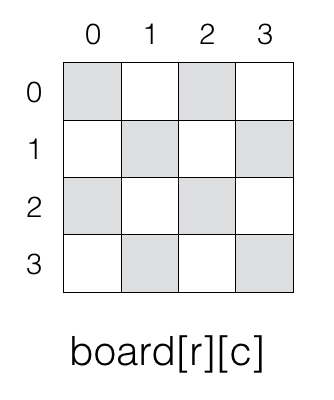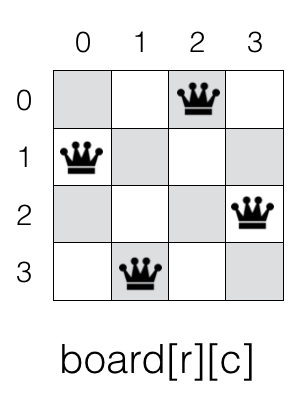# N Queen Problem

Backtracking Algorithm

In this tutorial we will learn about N Queen Problem using backtracking.

## Mission

If the chess board is of NxN size then our mission is to place N queens on the board such that each of them are at a safe position without getting attacked from other queens.

## Consider a 4x4 chess boardWe will start by placing a queen at position board i.e., row = 0 and column = 0.

We will place a queen in every column in such a way that no queen is able to attack another queen on the board.

At the end of this process we will have the following answerPlease note there can be more than one possible solution for a given N queen problem. And there is also a chance that a N queen problem will not have any solution.

For example, if N = 1 then solution is 1. When N = 2 then there is no solution as we can't put two queens on a chess board of size 2x2 without attacking each other.

## Code in C

``````
#include <stdio.h>

#define N 4

void display(int board[N][N]);
bool solveNQ(int board[N][N], int col);
bool isSafePos(int board[N][N], int row, int col);

void display(int board[N][N]) {
int r, c;
for(r = 0; r < N; r++) {
for(c = 0; c < N; c++) {
printf("%d ", board[r][c]);
}
printf("\n");
}
}

bool solveNQ(int board[N][N], int col) {
//variables
int i;

/*
if all the queens are placed then
the board is solved
*/
if(col >= N) {
return true;
}

/*
for the given column col
try placing the queen in all the
rows one by one to see if its
possible to place a queen in the
given column without dispute
*/
for(i = 0; i < N; i++) {
/*
check if queen can be placed
in cell board[i][col]
*/
if(isSafePos(board, i, col) == true) {
/*
the position is safe
so, we place a queen in the
cell board[i][col]
*/
board[i][col] = 1;

/*
now recursively place rest of
the queen
*/
if(solveNQ(board, col+1) == true) {
return true;
}

/*
if the queen is placed in cell
board[i][col] and it does not
leads to a solution then we reset
the cell i.e., backtrack
*/
board[i][col] = 0;
}
}

/*
if queen can&apost be placed in any row
for the given column col then there is
no solution
*/
return false;
}

bool isSafePos(int board[N][N], int row, int col) {
int r, c;

/*
this function will check if the position is safe
for the queen.

we need to check the rows and the diagonals
*/

/*
if there is a queen in the given row
then it is not a safe position
*/
for(c = 0; c < col; c++) {
if(board[row][c] == 1) {
return false;
}
}

/*
check upper diagonal
*/
for(r = row, c = col; r >= 0 && c >= 0; r--, c--) {
if(board[r][c] == 1) {
return false;
}
}

/*
check lower diagonal
*/
for (r = row, c = col; c >= 0 && r < N; r++, c--) {
if (board[r][c] == 1) {
return false;
}
}

return true;
}

int main(void) {
/*
board of size NxN
initially all the cells of the board
is set to 0

0 means there is no queen in the cell
1 means there is a queen in the cell
*/
int board[N][N] = {0};

if(solveNQ(board, 0) == false) {
printf("No solution!\n");
} else {
display(board);
}

return 0;
}
``````

## Output

``````
0 0 1 0
1 0 0 0
0 0 0 1
0 1 0 0
``````